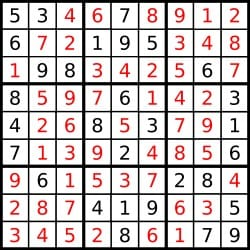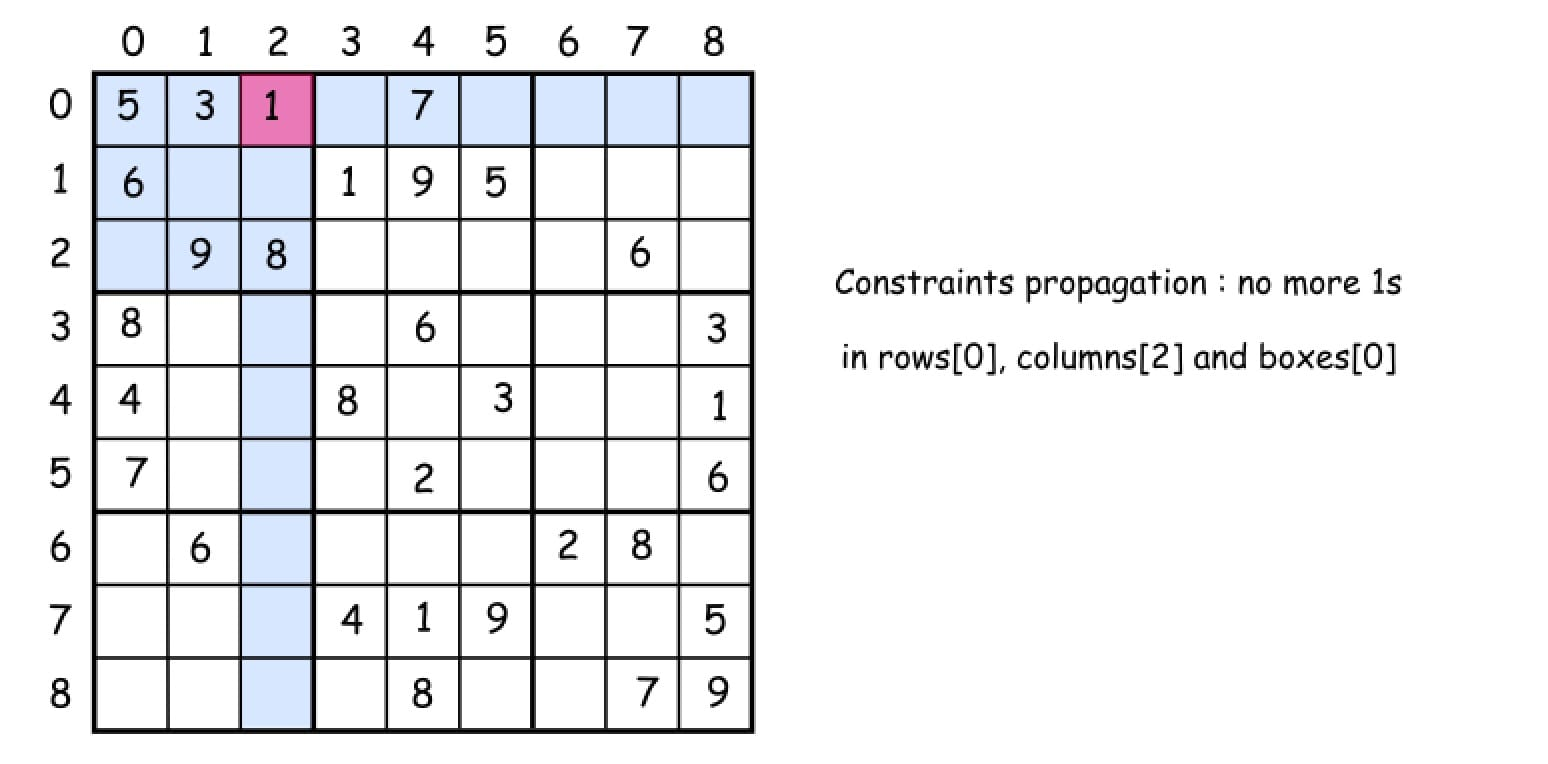# 37. Sudoku Solver

Reference: LeetCode
Difficulty: Hard

## Problem

Write a program to solve a Sudoku puzzle by filling the empty cells.

A sudoku solution must satisfy all of the following rules:

• Each of the digits 1-9 must occur exactly once in each row.
• Each of the digits 1-9 must occur exactly once in each column.
• Each of the the digits 1-9 must occur exactly once in each of the 9 3x3 sub-boxes of the grid.

Empty cells are indicated by the character '.'.Note:

• The given board contain only digits 1-9 and the character '.'.
• You may assume that the given Sudoku puzzle will have a single unique solution.
• The given board size is always 9x9.

## Analysis

### Brute-Force

Each cell could be filled number from 1 to 9 and then check validity. There could be $9^81$ operations to do, where $81$ is the number of cells to fill.

### Backtracking

From LeetCode solution section:The basic idea is to do some pre-processing. We calculate many sets in advance to make operations in backtracking faster. In backtracking, we examine each empty position’s all possibilities, which could be calculated by set operations.

Note:

• Set Operations (the modification):
• Union: A.addAll(B)
• Intersection: A.retainAll(B)
• Difference: A.removeAll(B)

Time: $O(1)$
Space: $O(1)$

CommentJunhao Wang
a software engineering cat